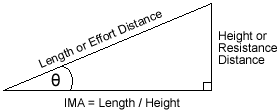An inclined plane is a plane surface set at an angle, other than a right angle, against a horizontal surface. An inclined plane is one of the commonly-recognized simple machines.Simple machine

## Contents

The inclined plane permits one to overcome a large resistance by applying a relatively small force through a longer distance than the load is to be raised.

In civil engineering the slope (ratio of rise/run) is often referred to as a grade or gradient.

## Examples of Inclined Planes

Examples of inclined planes are ramps, sloping roads, chisels, hatchets, plows, air hammers, carpenter's planes and wedges. The most canonical example of an inclined plane is a sloped surface; for example a roadway to bridge a height difference.

### Ramps

The ramp makes it easier to move a physical body vertically by extending the distance traveled horizontally (run) to achieve the desired elevation change (rise). By changing the angle of the ramp one can usefully vary the force necessary to raise or lower a load.

##Ramps are used as alternatives for stairways, for wheelchairs, buggies and shopping carts. Ramps may zigzag. There are also moving ramps such as the mobile staircases by which passengers board and leave an aircraft.

For example: A wagon trail on a steep hill will often traverse back and forth to reduce the gradient experienced by a team pulling a heavily loaded wagon. This same technique is used today in modern freeways which travel through steep mountain passes. Some steep passes have separate truck routes that reduce the grade by winding along a separate path to rejoin the main route after a particularly steep section is past while smaller automobiles take the straighter steeper route with a resulting savings in time.

Other simple machines based on the inclined plane include the blade, in which two inclined planes placed back to back allow the two parts of the cut object to move apart using less force than would be needed to pull them apart in opposite directions.

## History

The ramp or inclined plane was useful in building early stone edifices, roads and aqueducts. It was also used for military assault of fortified positions.

Experiments with inclined planes helped early physicists such as Galileo Galilei quantify the behavior of nature with respect to gravity, mass, acceleration, etc.

Detailed understanding of inclined planes and their use helped lead to the understanding of how vector quantities such as forces can be usefully decomposed and manipulated mathematically. This concept of superposition and decomposition is critical in many modern fields of science, engineering, and technology.

## Physics Incline Plane Problem

Incline plane is an interesting subject in Physics, because of its effect on the object that is on the incline plane (see picture below). In Physics, it is important to analyze the forces that is acting on the block (Newton's Laws), and to create an equation out of the analysis.

For example, the picture below has 3 force vectors (neglecting air resistance and the mass sliding down the incline plane).

The first vector is the normal force, which is always perpendicular to the ground. It is also the force that is stopping the object from accelerating at gravity's full acceleration (On Earth, gravity acceleration is 9.8 meters/seconds squared).

The second vector is gravity, the planet's attractive force. Within the gravity vector, there are two components, horizontal and vertical force in the incline plane perspective. As you can see the angle is on the lower left corner.

What happens if we make that angle zero degrees? The plane will be horizontal and the only force acting on it is gravity (pointing downwards)and normal force (pointing upwards). The only Trigonometry function that makes zero degree = 1 is Cosine, so cosine must be the vertical force. With this fact, we can go back to the incline plane and use the cosine function to find the vertical force, and the sine function to find the horizontal force.

The incline plane is also in contact with the massed block, and the contact causes friction. So the third vector is friction. Since the block is sliding downwards, the friction vector is going the opposite of the horizontal force of gravity.

Taking all these vectors into consideration, we can say the normal force and the vertical component of gravity balances out, because it is not accelerating upwards nor downwards, but side ways. And since, friction is going the opposite direction of the horizontal gravity force, the whole equation is. The mA part is derived from Newton's laws of motion.

Key:

N = Normal Force that is perpendicular to the plane

m = Mass of object

g = Gravity of the planet

θ (theta) = Angle of elevation of the plane, which is measured from the horizontal

f = Frictional Force of the inclined plane

Special cases: WhenmgSinθ = 0 and mgCosθ = 1 which is a regular flat surface.

When WhenmgSinθ = 1 and mgCosθ = 0 which means the object is falling straight down.

mgSinθ - f = Net Force of the object on the inclined plane.

 This page uses Creative Commons Licensed content from Wikipedia (view authors).